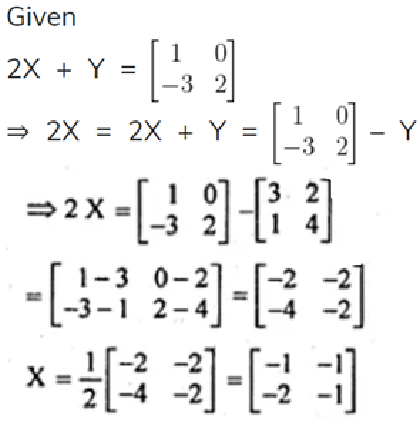# ML Aggarwal Solutions for Class 10 Maths Chapter 8 Matrices

ML Aggarwal Solutions For Class 10 Maths Chapter 8 Matrices consists of accurate solutions, which help the students to quickly complete their homework and prepare well for the exams. These Solutions of ML Aggarwal provide students an advantage with practical questions. Each step in the solution is explained to match students’ understanding. To score good marks in Class 10 Mathematics examination, it is advised they solve questions provided at the end of each chapter in the ML Aggarwal textbooks for Class 10. This chapter deals with matrices, types of matrices and product of matrices. A rectangular array of m x n numbers (real or complex) in the form of m horizontal lines (called rows) and n vertical lines (called columns), is called a matrix of order m by n, written as m x n matrix. Such an array is enclosed by [ ] or ( ). This chapter of ML Aggarwal Solutions for Class 10 contains three exercises with chapter test. These solutions provided by BYJU’S cover all these concepts, with detailed explanations.

## ML Aggarwal Solutions for Class 10 Maths Matrices:-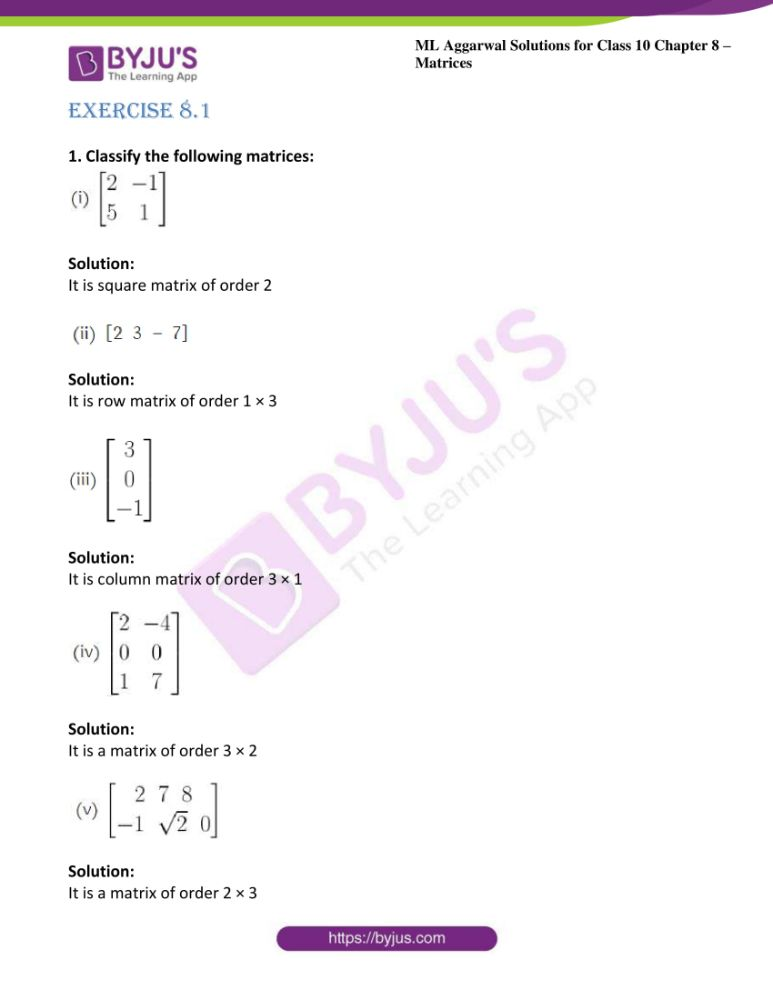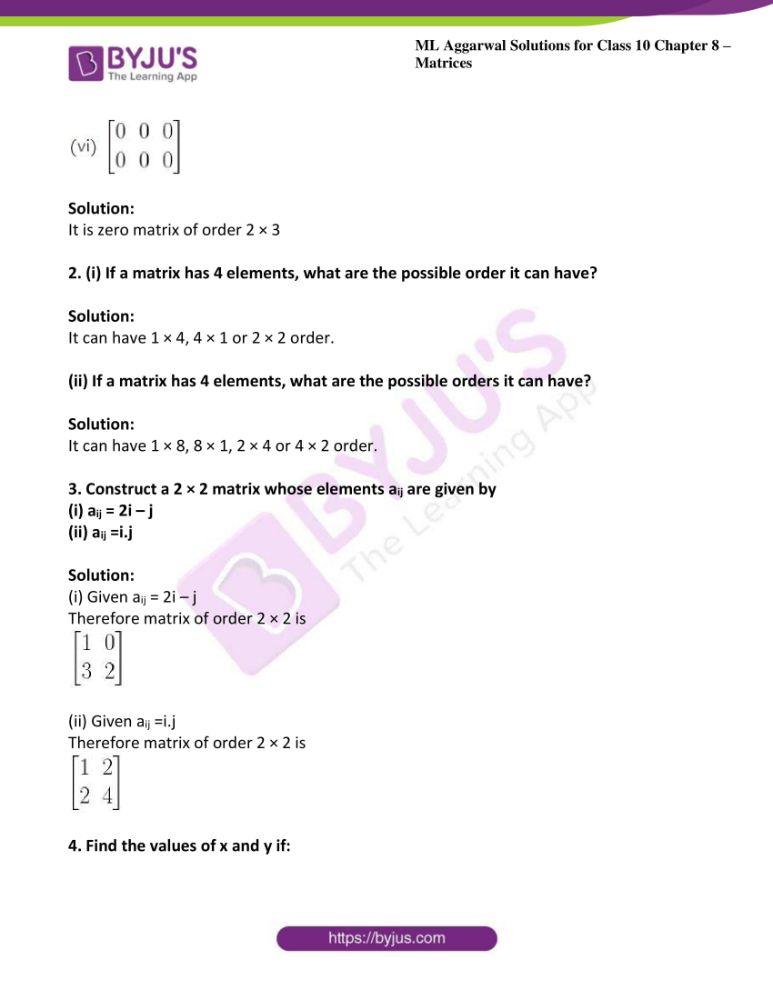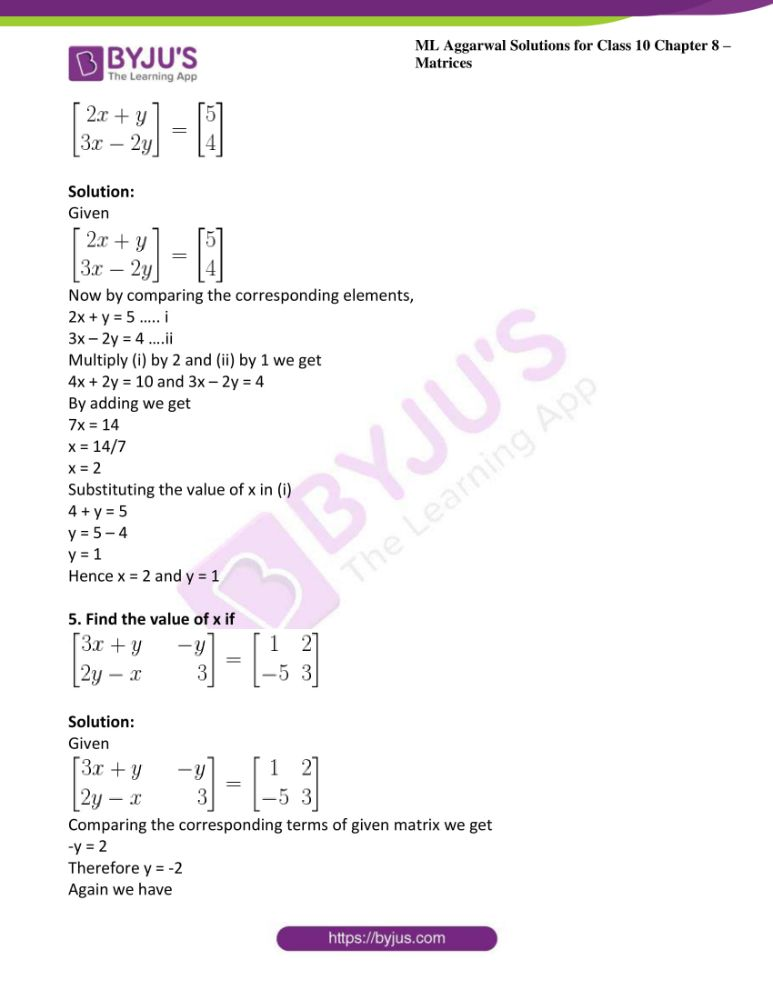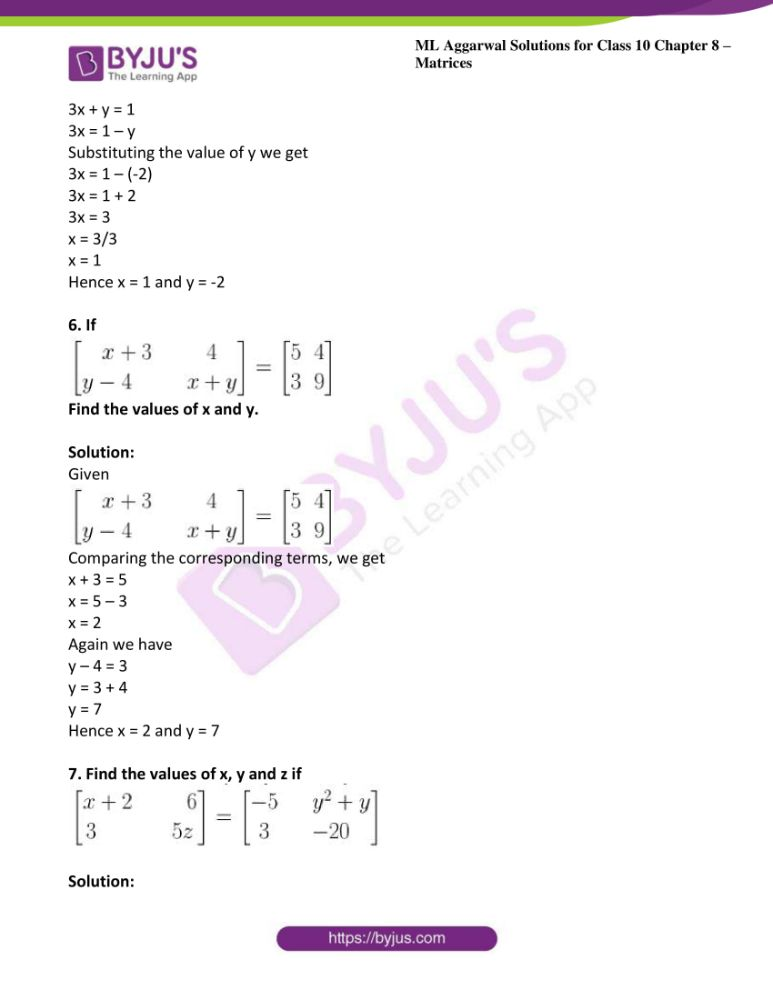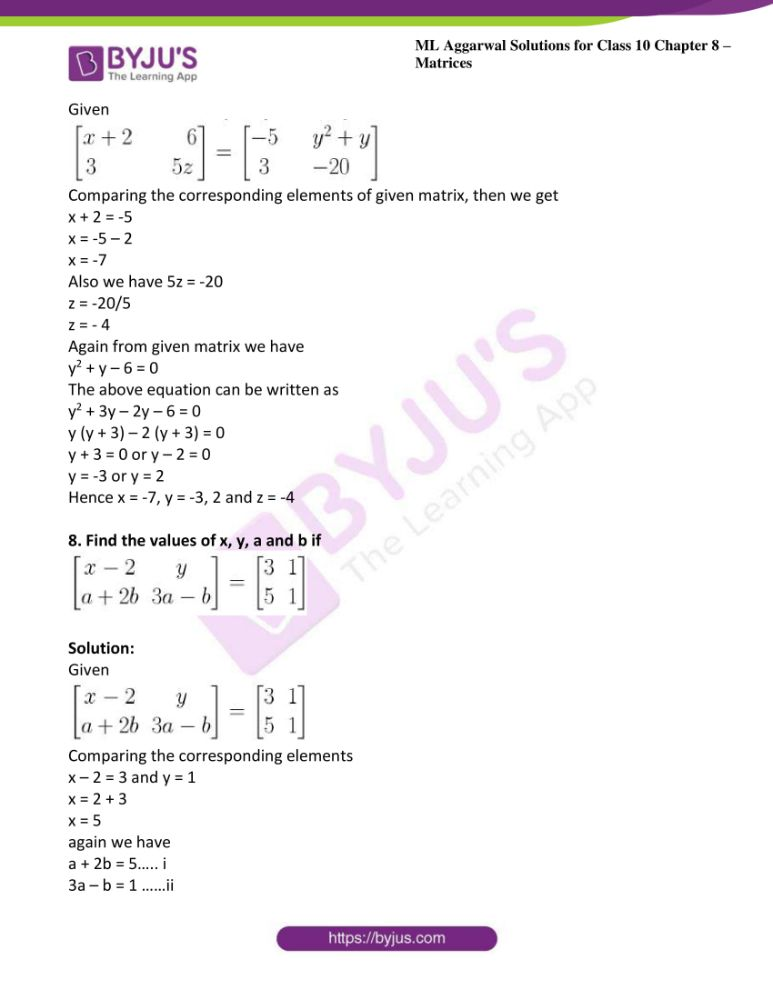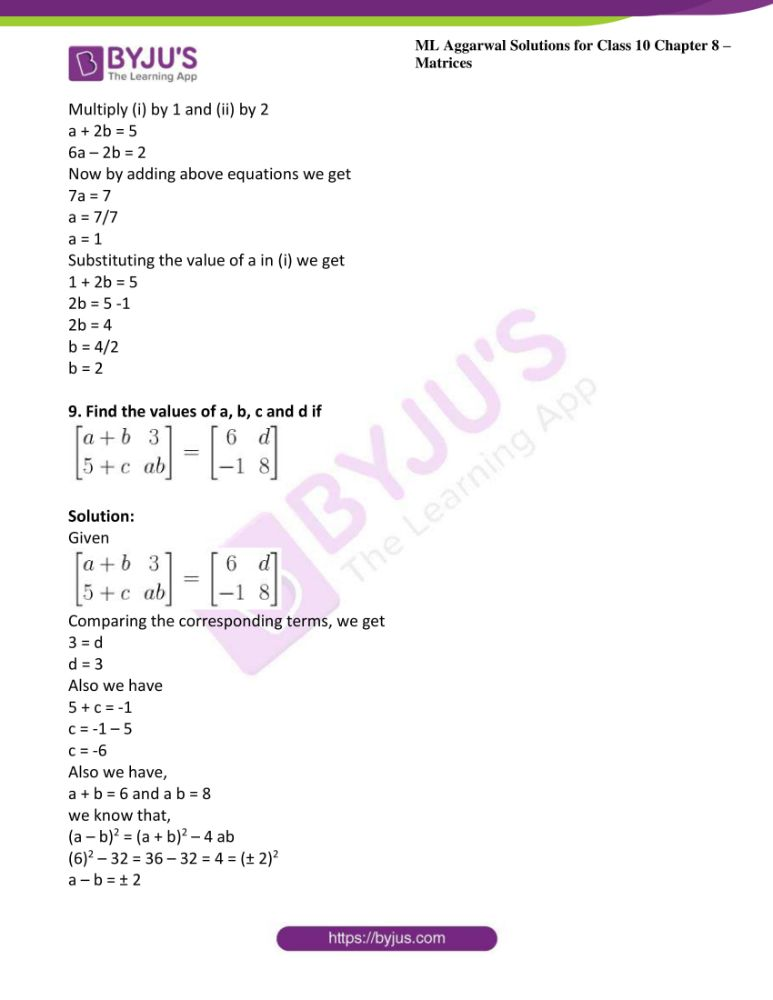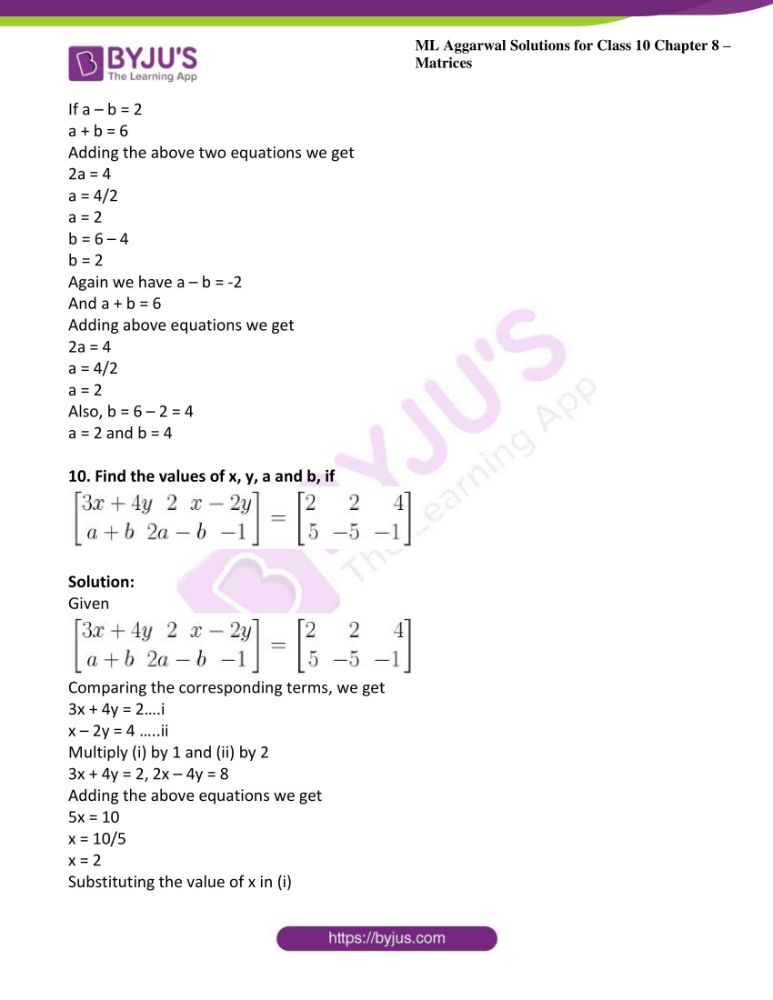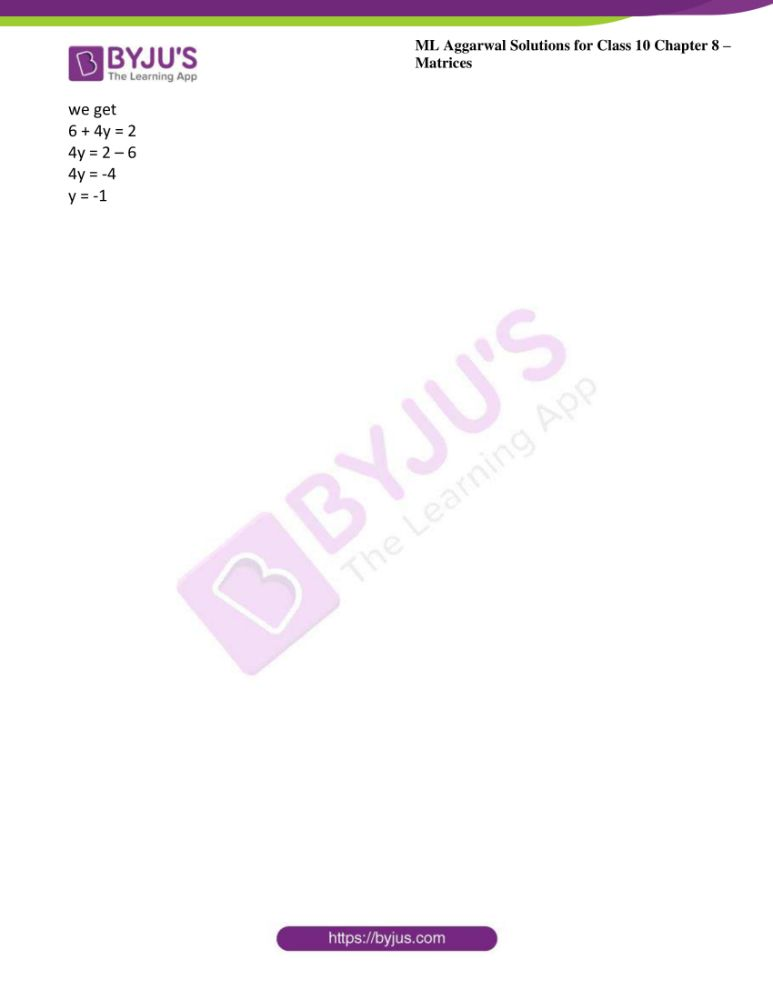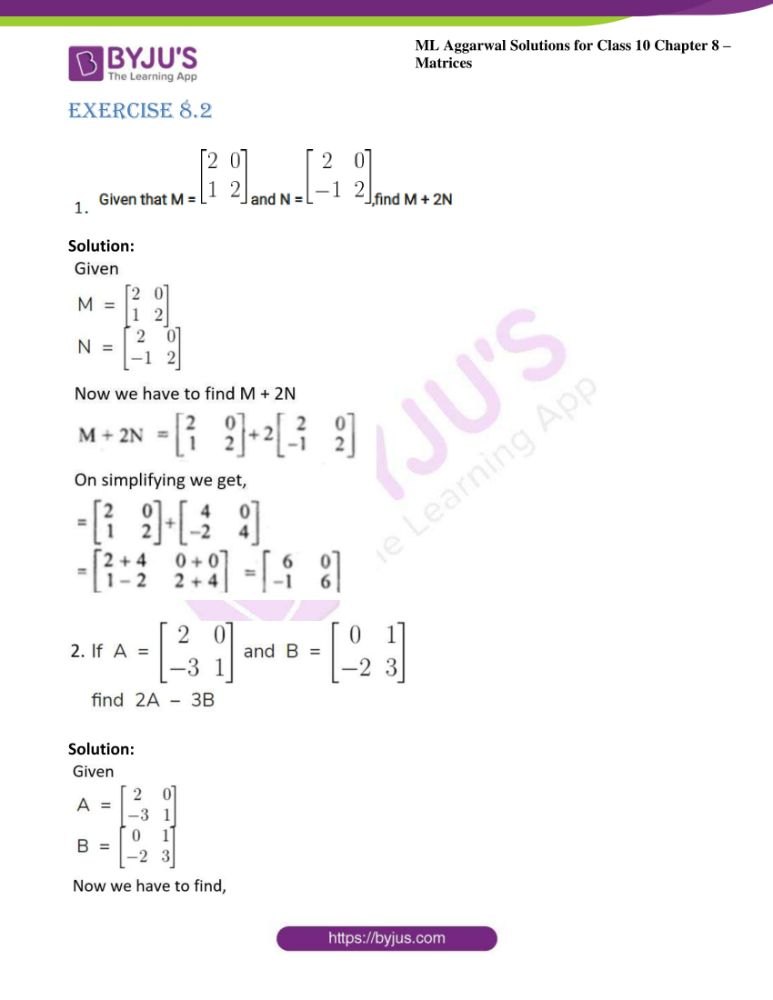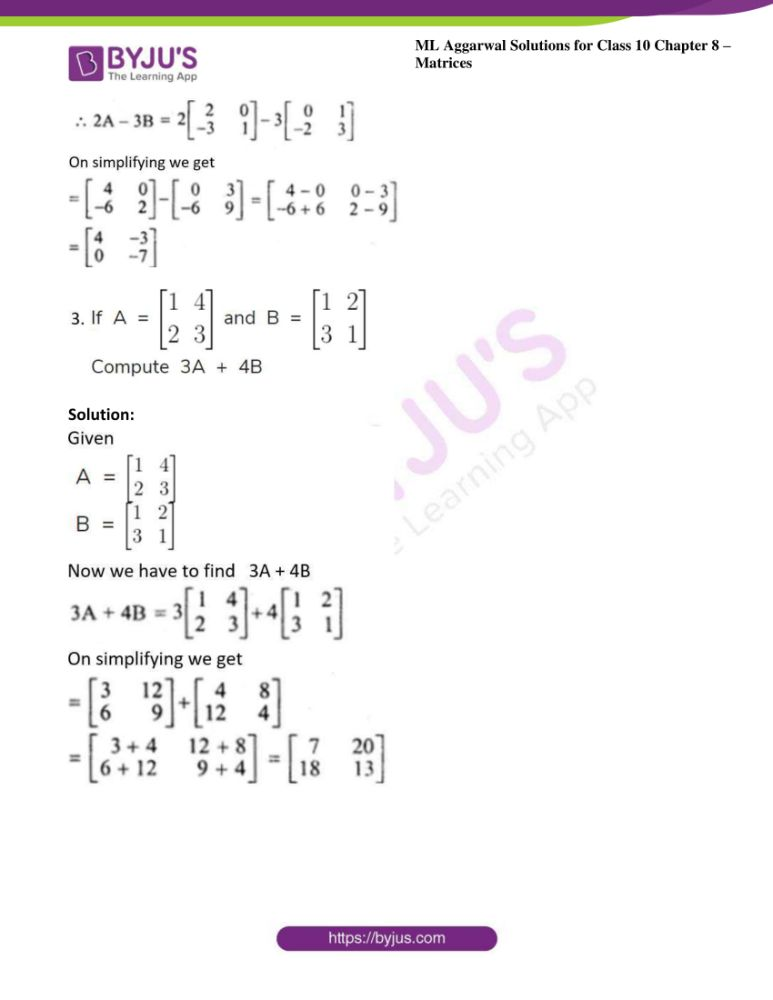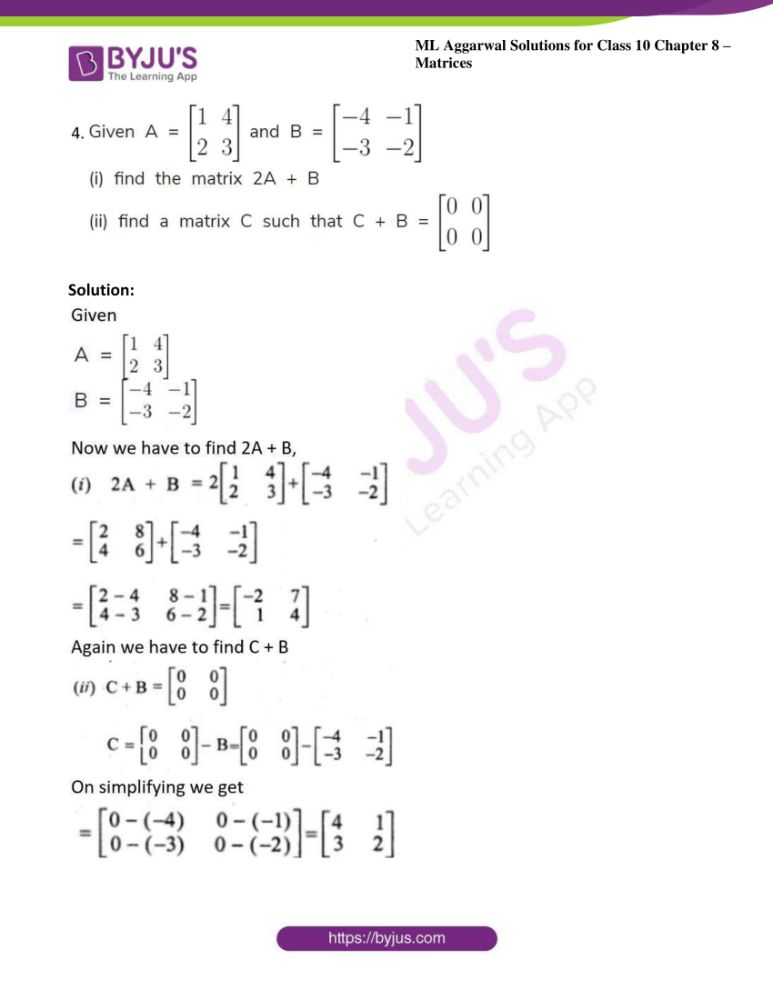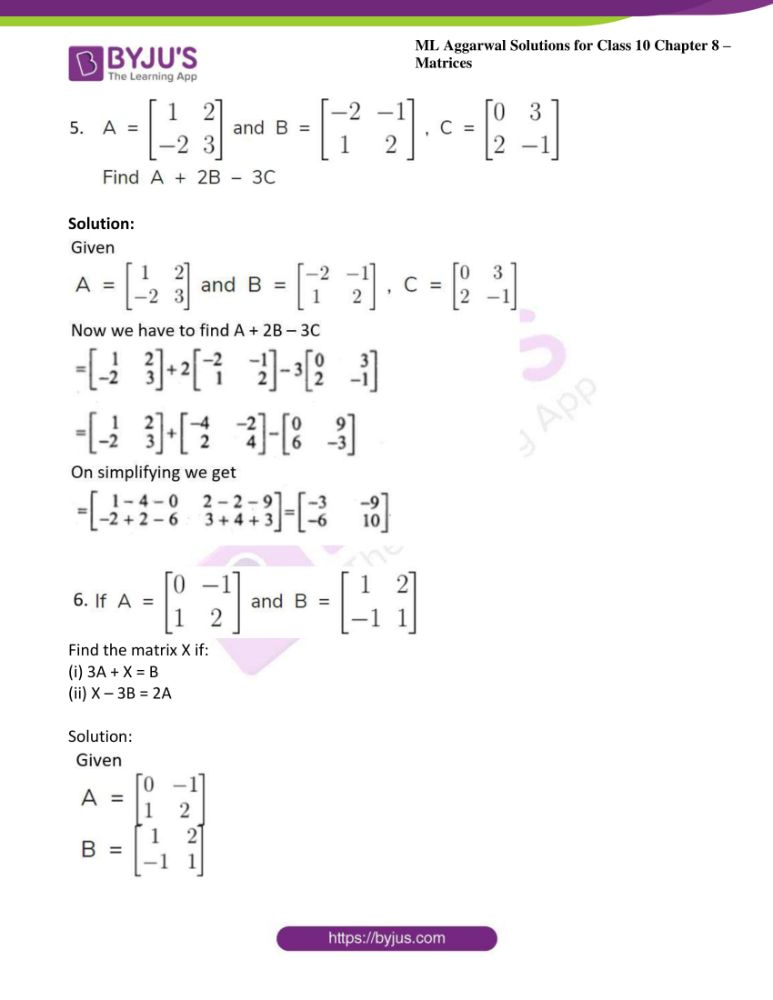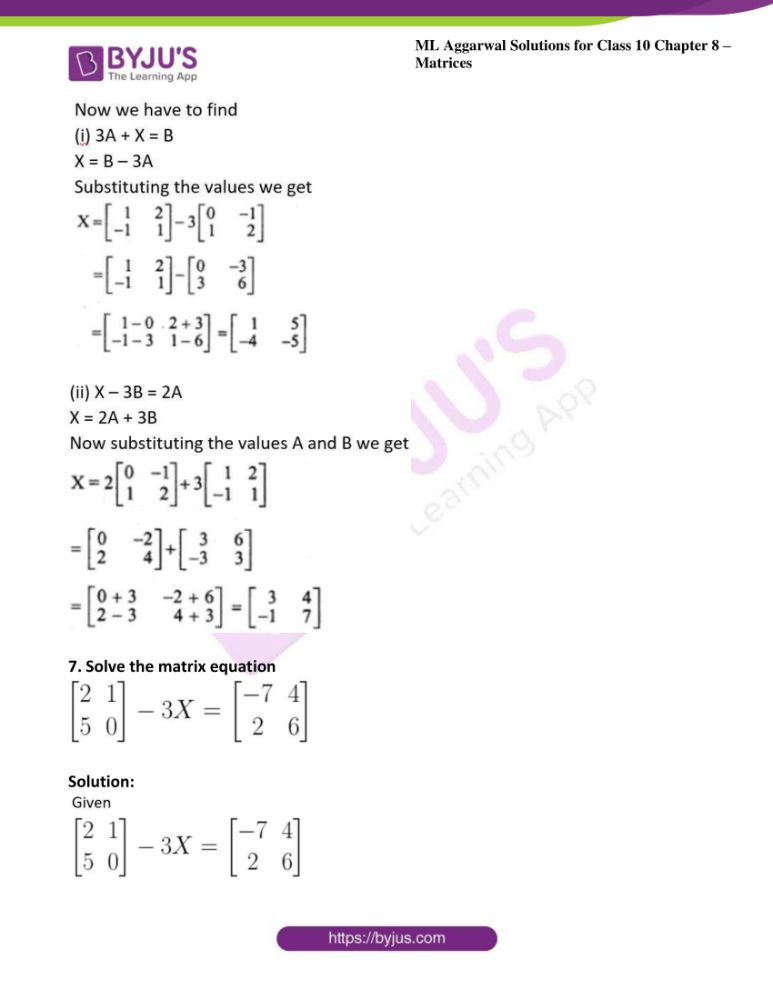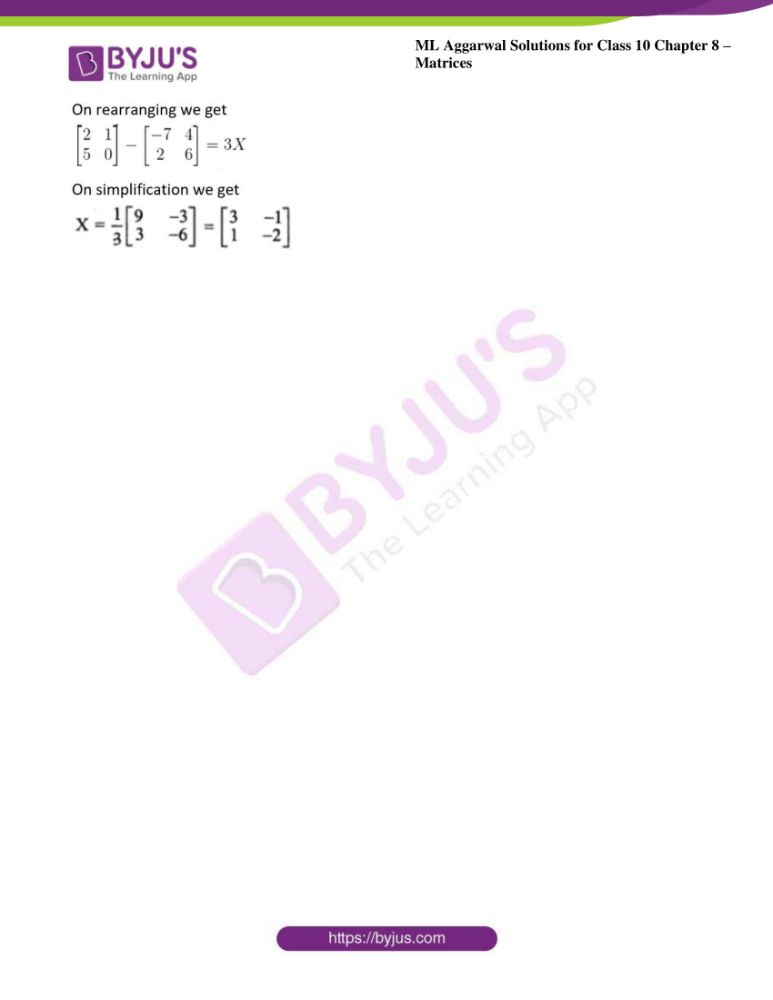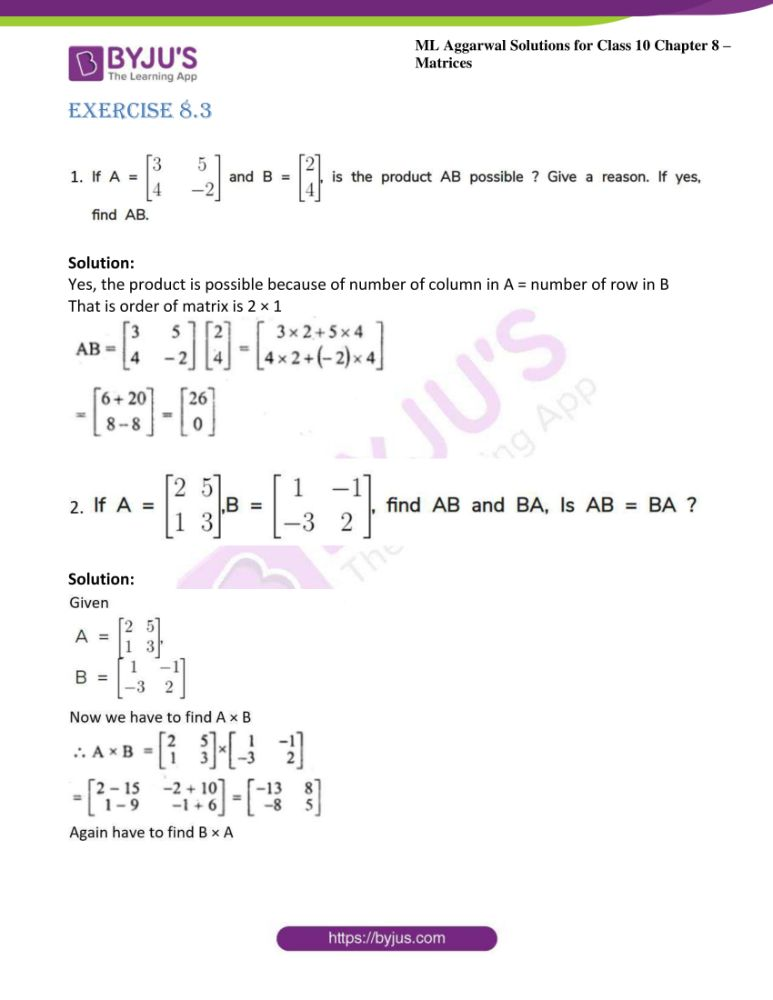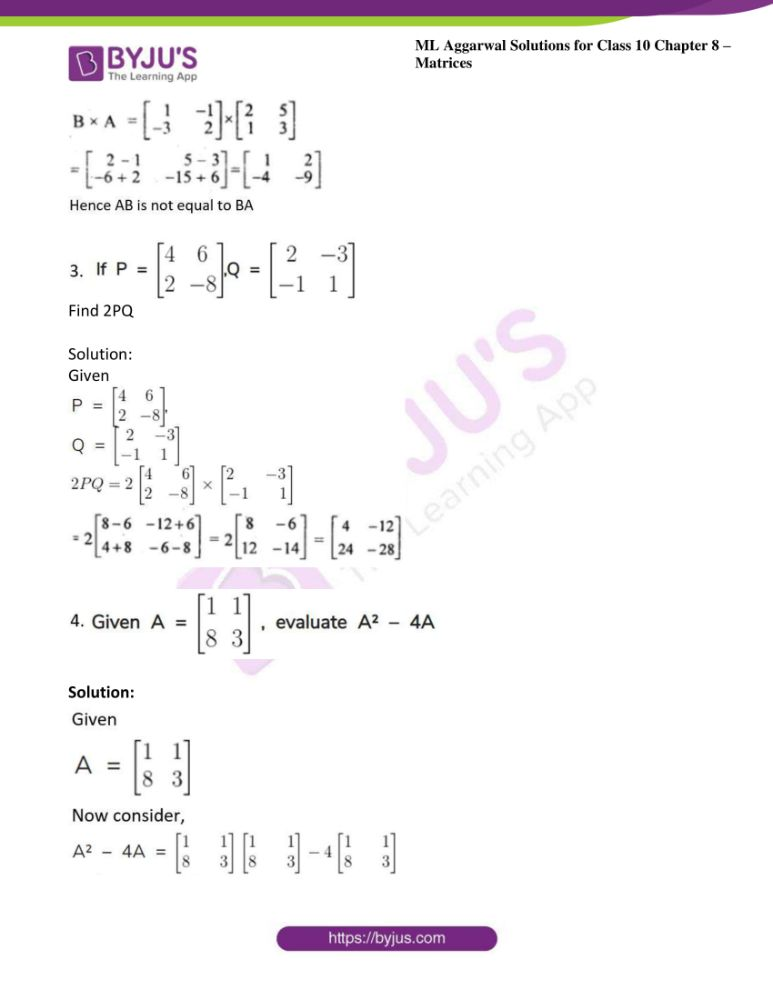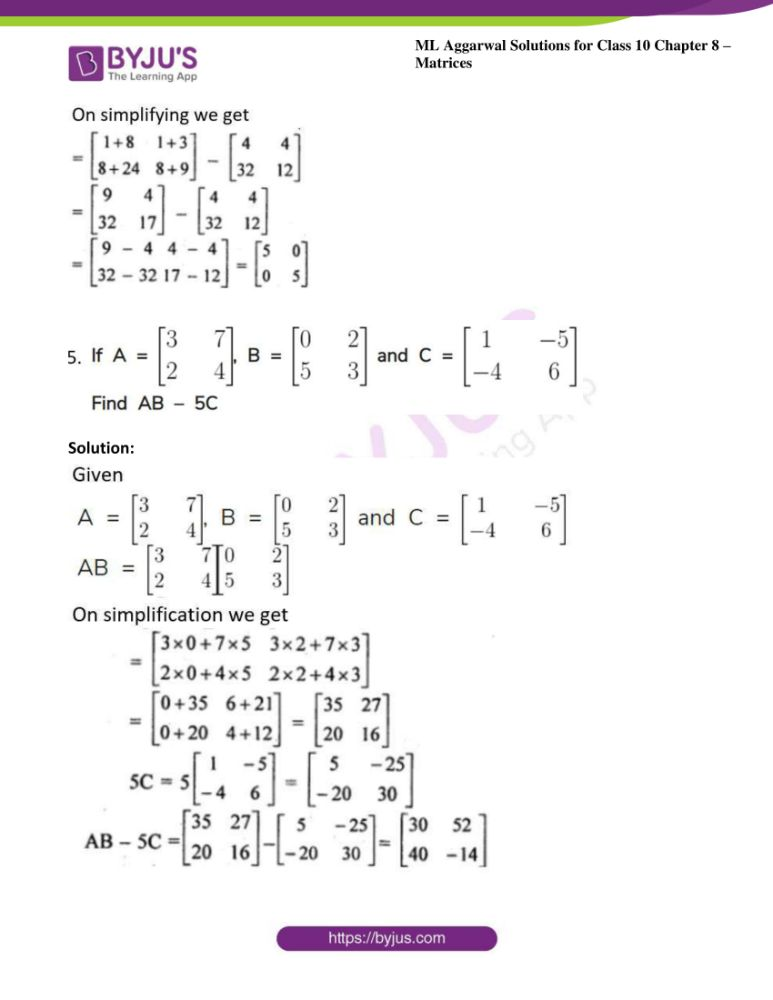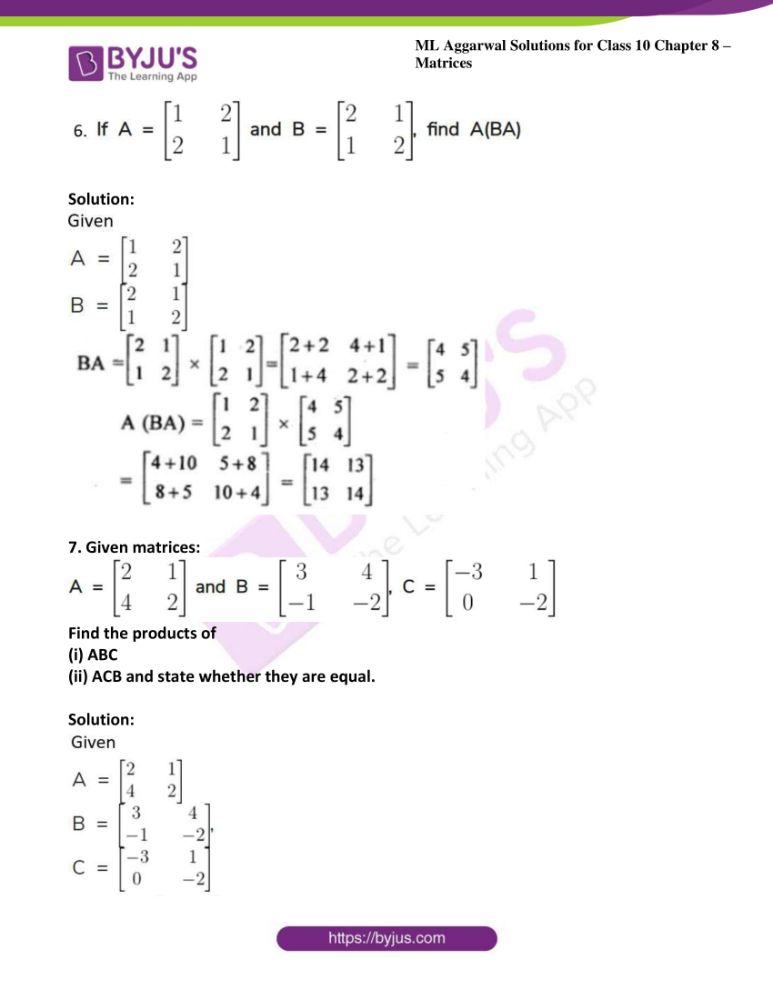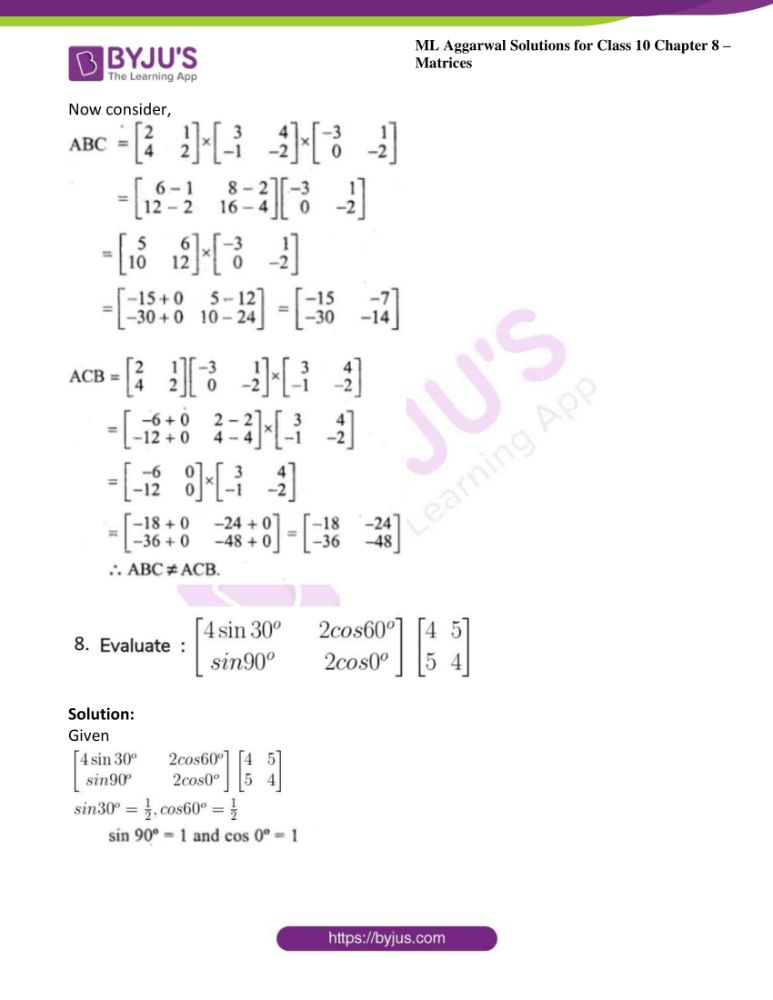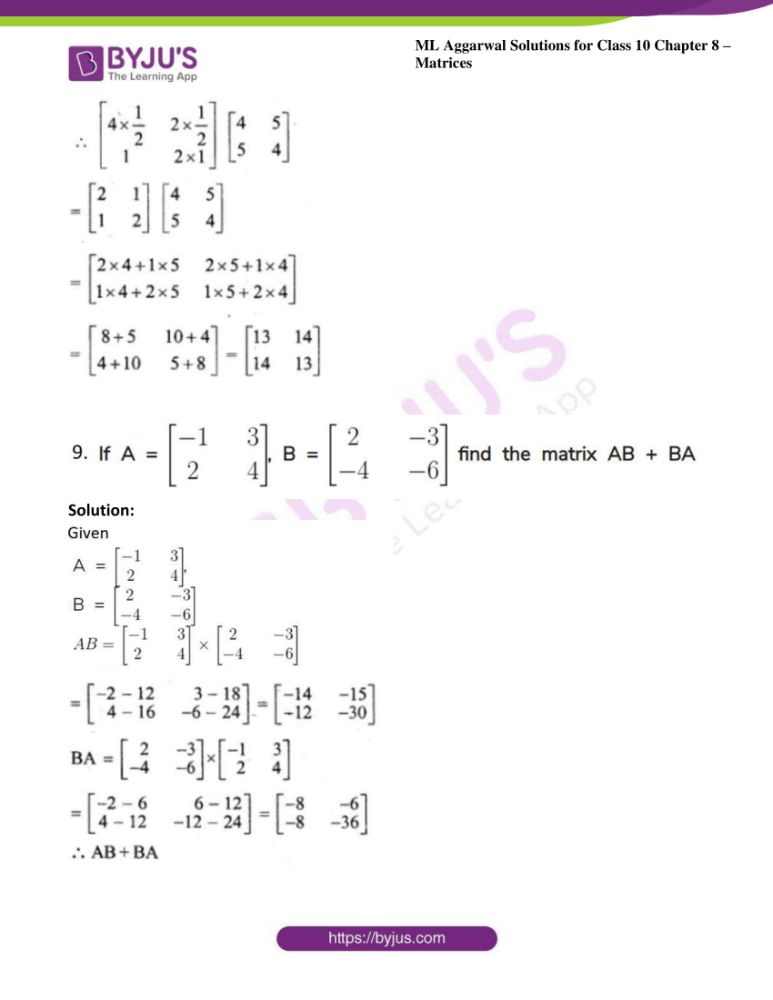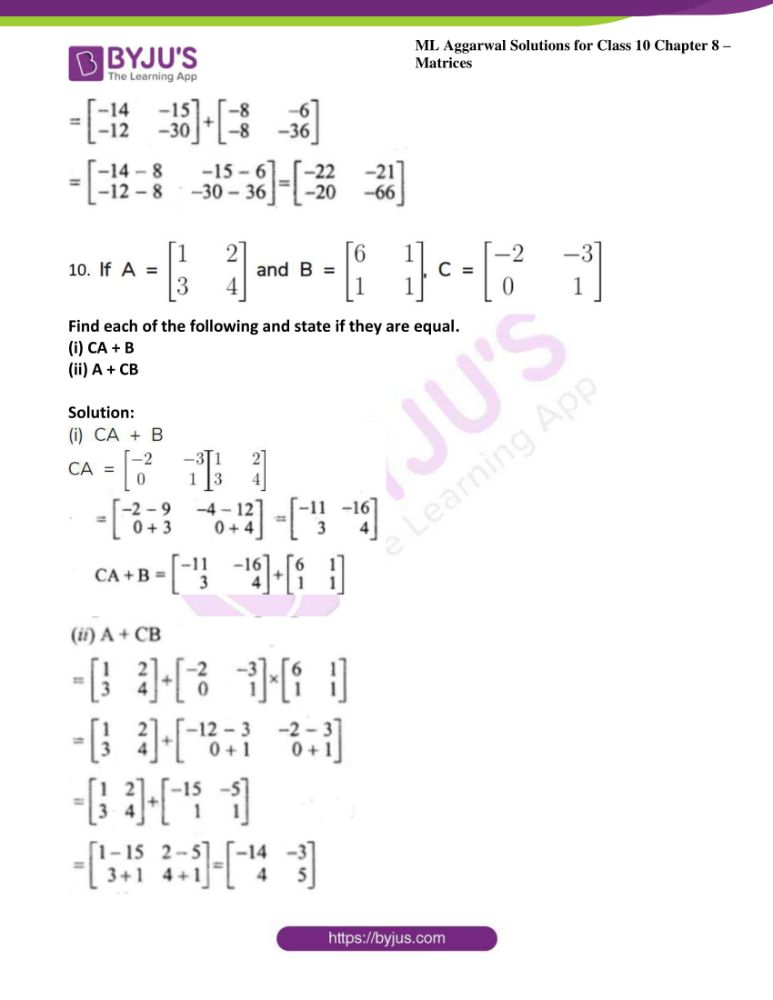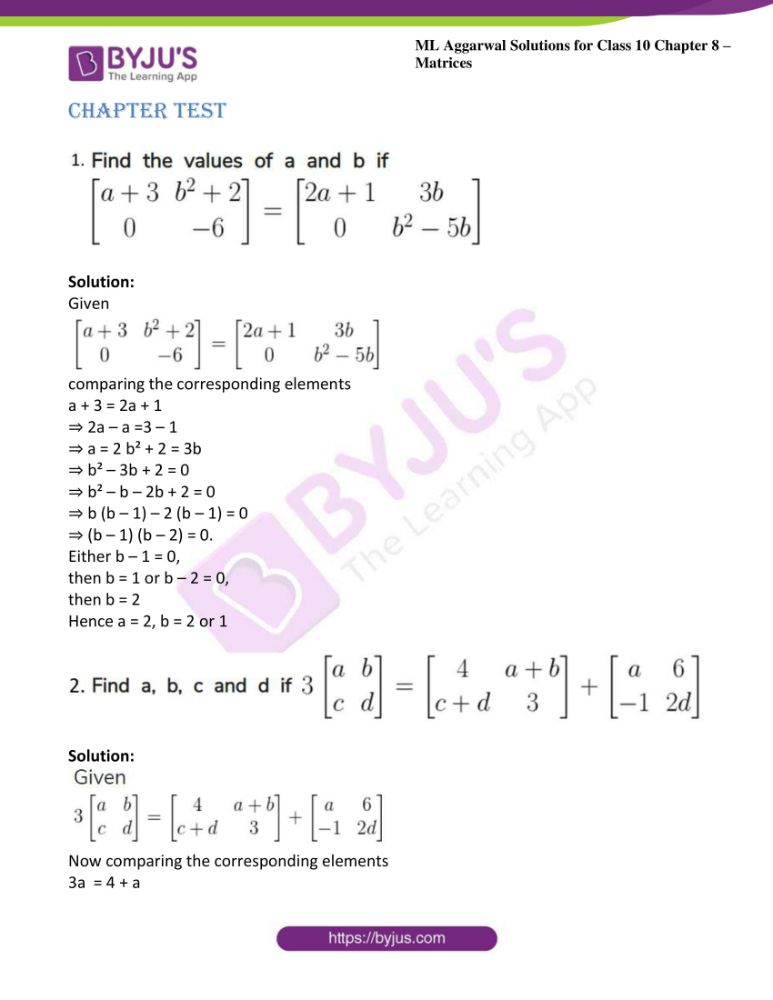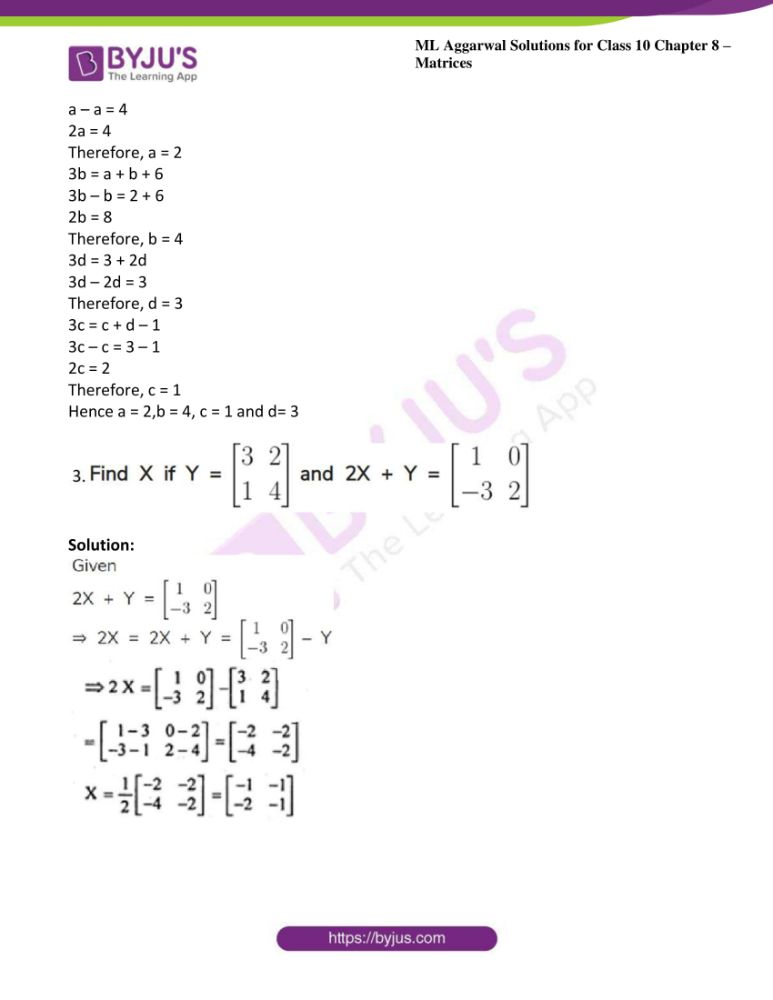### Access answers to ML Aggarwal Solutions for Class 10 Maths Chapter 8 – Matrices

Exercise 8.1

1. Classify the following matrices: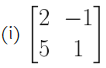Solution:

It is square matrix of order 2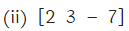Solution:

It is row matrix of order 1 × 3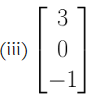Solution:

It is column matrix of order 3 × 1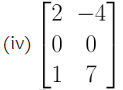Solution:

It is a matrix of order 3 × 2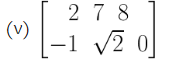Solution:

It is a matrix of order 2 × 3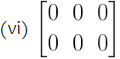Solution:

It is zero matrix of order 2 × 3

2. (i) If a matrix has 4 elements, what are the possible order it can have?

Solution:

It can have 1 × 4, 4 × 1 or 2 × 2 order.

(ii) If a matrix has 4 elements, what are the possible orders it can have?

Solution:

It can have 1 × 8, 8 × 1, 2 × 4 or 4 × 2 order.

3. Construct a 2 × 2 matrix whose elements aij are given by

(i) aij = 2i – j

(ii) aij =i.j

Solution:

(i) Given aij = 2i – j

Therefore matrix of order 2 × 2 is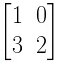(ii) Given aij =i.j

Therefore matrix of order 2 × 2 is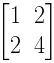4. Find the values of x and y if: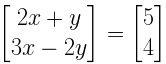Solution:

GivenNow by comparing the corresponding elements,

2x + y = 5 ….. i

3x – 2y = 4 ….ii

Multiply (i) by 2 and (ii) by 1 we get

4x + 2y = 10 and 3x – 2y = 4

7x = 14

x = 14/7

x = 2

Substituting the value of x in (i)

4 + y = 5

y = 5 – 4

y = 1

Hence x = 2 and y = 1

5. Find the value of x if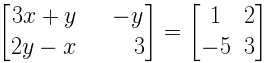Solution:

GivenComparing the corresponding terms of given matrix we get

-y = 2

Therefore y = -2

Again we have

3x + y = 1

3x = 1 – y

Substituting the value of y we get

3x = 1 – (-2)

3x = 1 + 2

3x = 3

x = 3/3

x = 1

Hence x = 1 and y = -2

6. If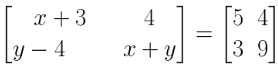Find the values of x and y.

Solution:

GivenComparing the corresponding terms, we get

x + 3 = 5

x = 5 – 3

x = 2

Again we have

y – 4 = 3

y = 3 + 4

y = 7

Hence x = 2 and y = 7

7. Find the values of x, y and z if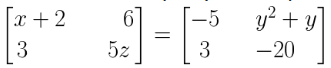Solution:

GivenComparing the corresponding elements of given matrix, then we get

x + 2 = -5

x = -5 – 2

x = -7

Also we have 5z = -20

z = -20/5

z = – 4

Again from given matrix we have

y2 + y – 6 = 0

The above equation can be written as

y2 + 3y – 2y – 6 = 0

y (y + 3) – 2 (y + 3) = 0

y + 3 = 0 or y – 2 = 0

y = -3 or y = 2

Hence x = -7, y = -3, 2 and z = -4

8. Find the values of x, y, a and b if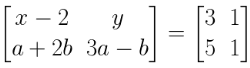Solution:

GivenComparing the corresponding elements

x – 2 = 3 and y = 1

x = 2 + 3

x = 5

again we have

a + 2b = 5….. i

3a – b = 1 ……ii

Multiply (i) by 1 and (ii) by 2

a + 2b = 5

6a – 2b = 2

Now by adding above equations we get

7a = 7

a = 7/7

a = 1

Substituting the value of a in (i) we get

1 + 2b = 5

2b = 5 -1

2b = 4

b = 4/2

b = 2

9. Find the values of a, b, c and d if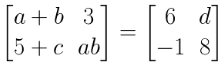Solution:

GivenComparing the corresponding terms, we get

3 = d

d = 3

Also we have

5 + c = -1

c = -1 – 5

c = -6

Also we have,

a + b = 6 and a b = 8

we know that,

(a – b)2 = (a + b)2 – 4 ab

(6)2 – 32 = 36 – 32 = 4 = (± 2)2

a – b = ± 2

If a – b = 2

a + b = 6

Adding the above two equations we get

2a = 4

a = 4/2

a = 2

b = 6 – 4

b = 2

Again we have a – b = -2

And a + b = 6

2a = 4

a = 4/2

a = 2

Also, b = 6 – 2 = 4

a = 2 and b = 4

10. Find the values of x, y, a and b, if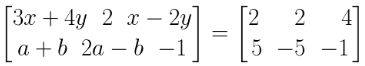Solution:

GivenComparing the corresponding terms, we get

3x + 4y = 2….i

x – 2y = 4 …..ii

Multiply (i) by 1 and (ii) by 2

3x + 4y = 2, 2x – 4y = 8

Adding the above equations we get

5x = 10

x = 10/5

x = 2

Substituting the value of x in (i)

we get

6 + 4y = 2

4y = 2 – 6

4y = -4

y = -1

Exercise 8.2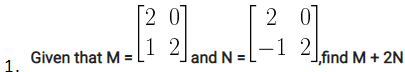Solution: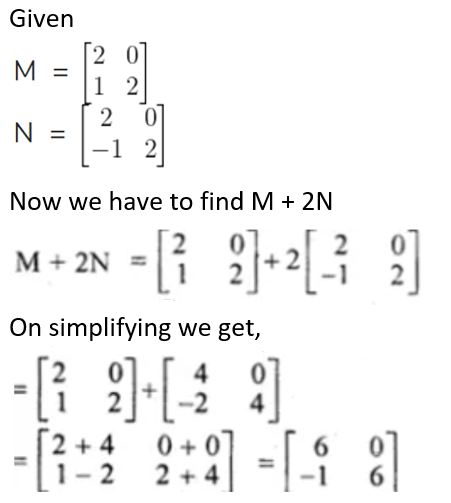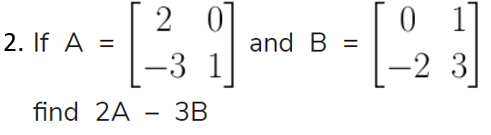Solution: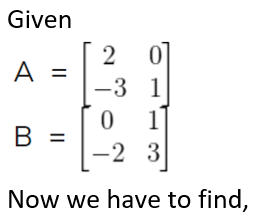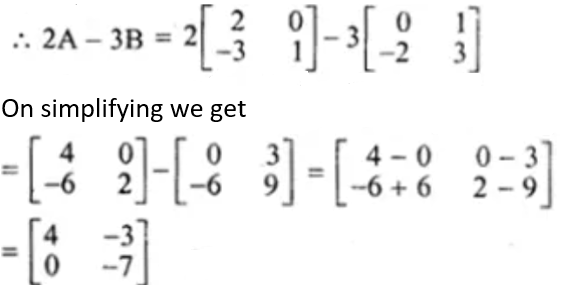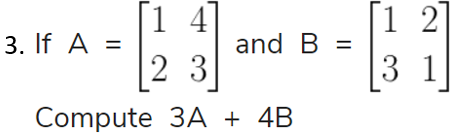Solution: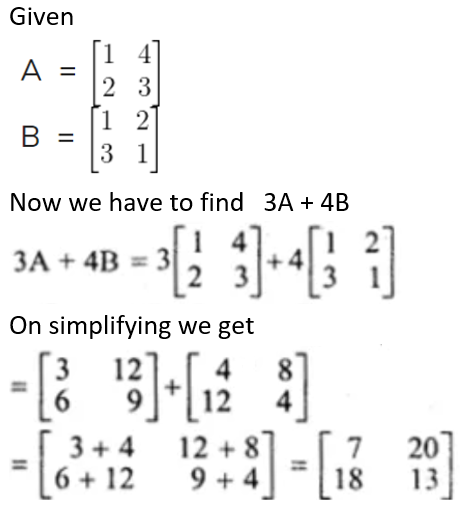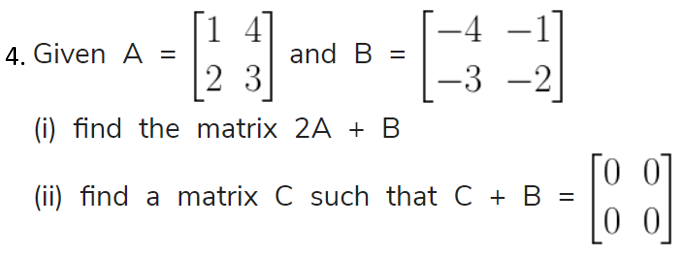Solution: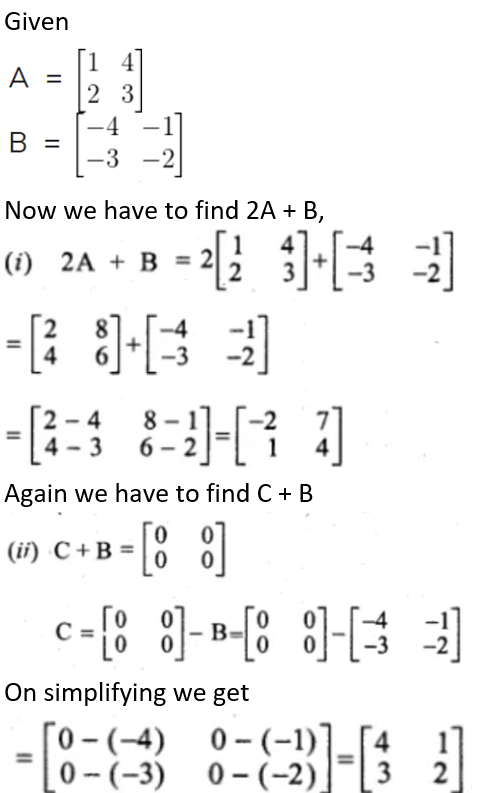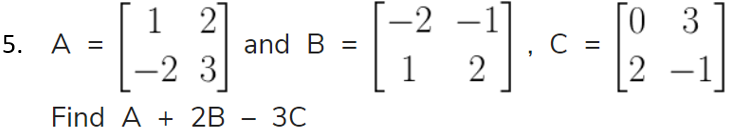Solution: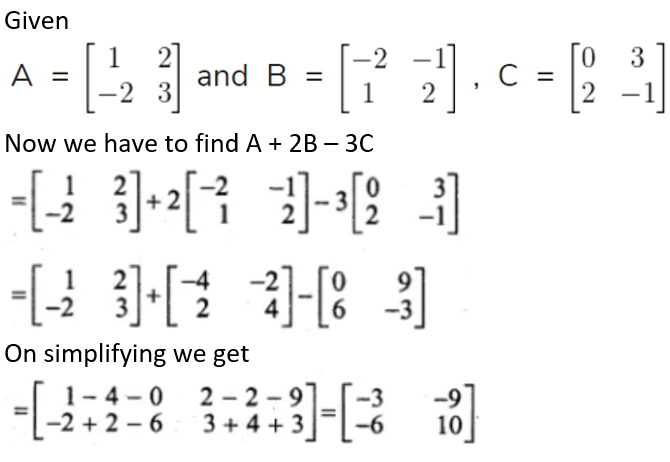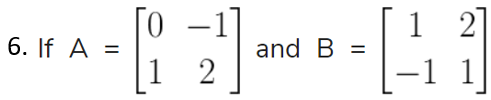Find the matrix X if:

(i) 3A + X = B

(ii) X – 3B = 2A

Solution: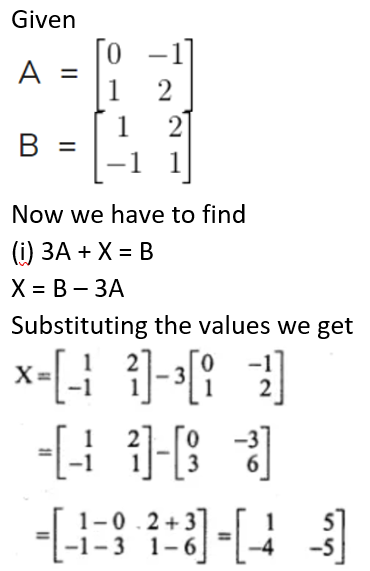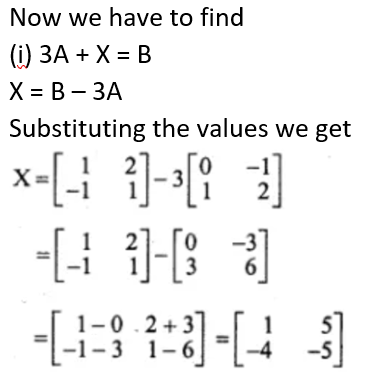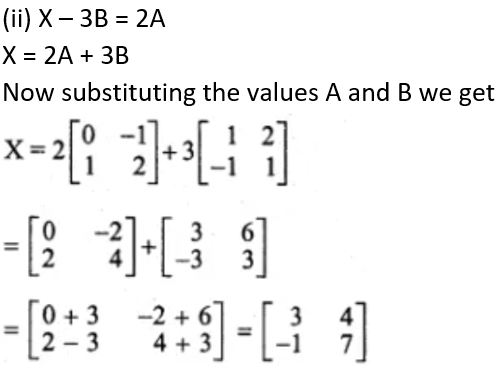7. Solve the matrix equation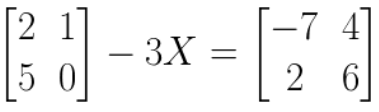Solution: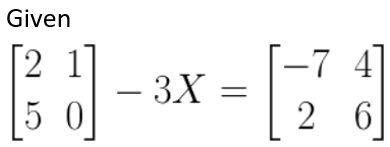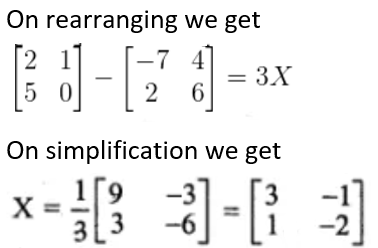Exercise 8.3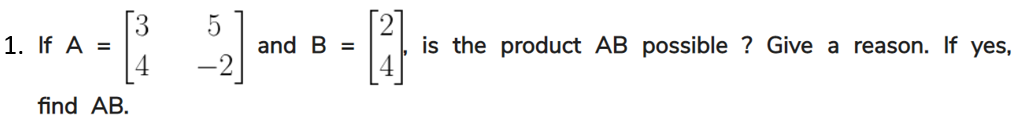Solution:

Yes, the product is possible because of number of column in A = number of row in B

That is order of matrix is 2 × 1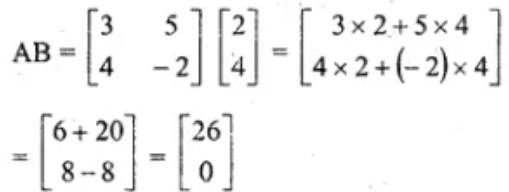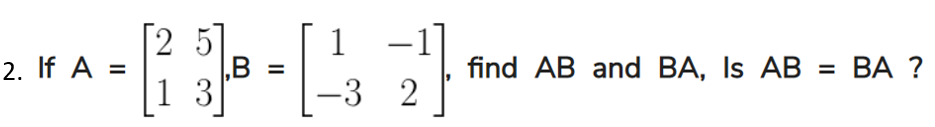Solution: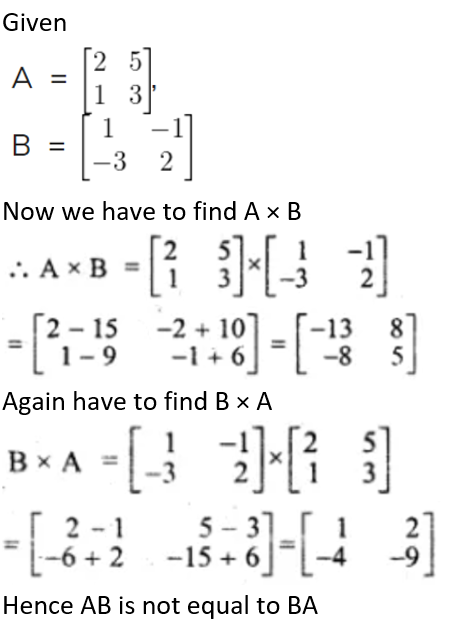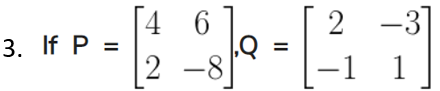Find 2PQ

Solution:

Given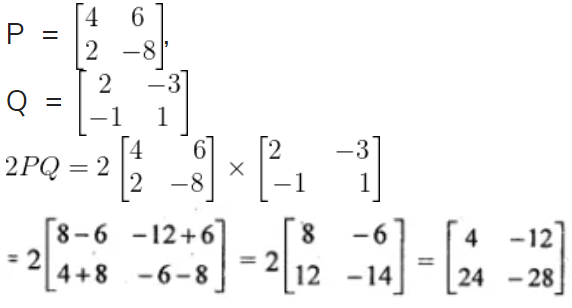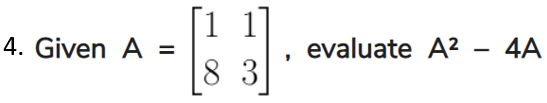Solution: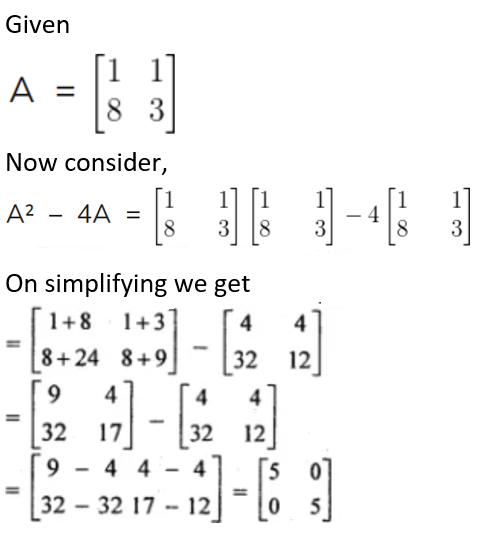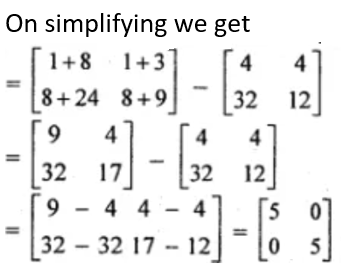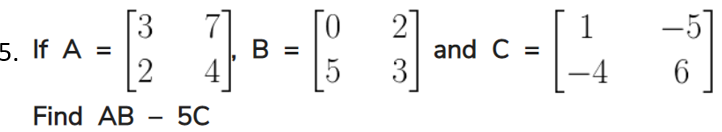Solution: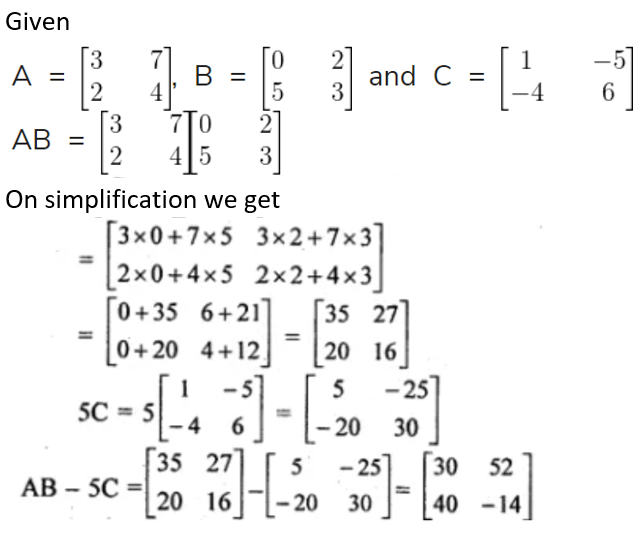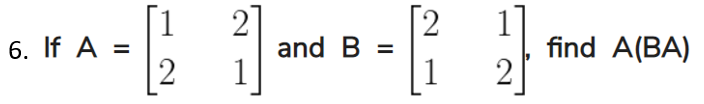Solution: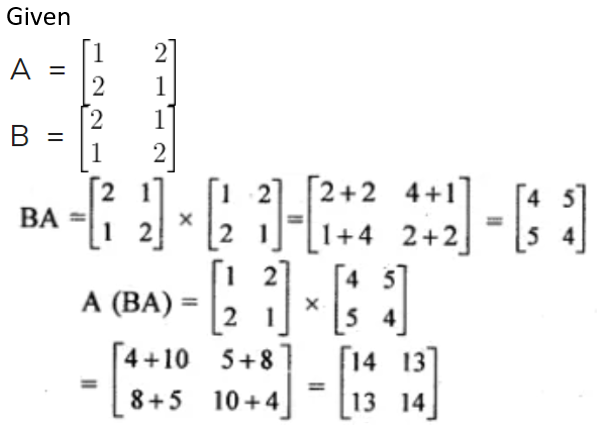7. Given matrices: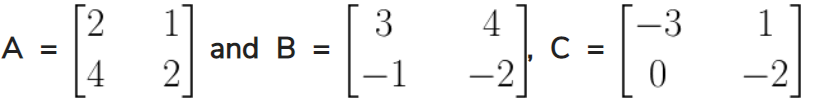Find the products of

(i) ABC

(ii) ACB and state whether they are equal.

Solution: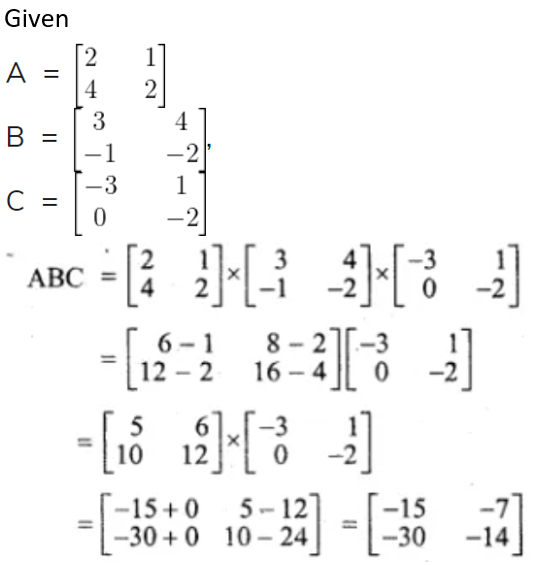Now consider,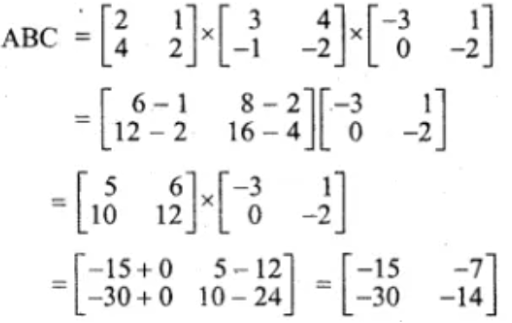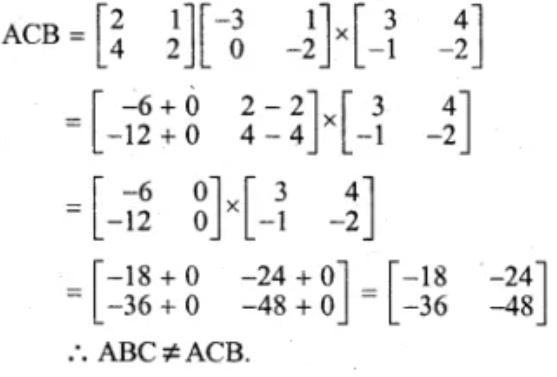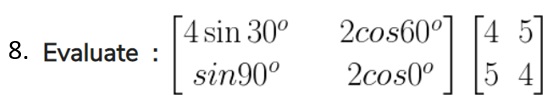Solution:

Given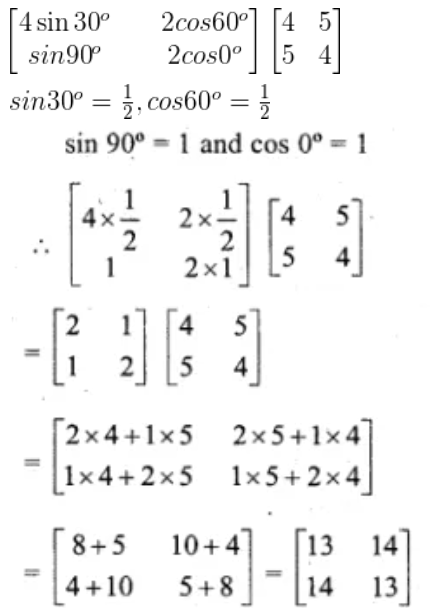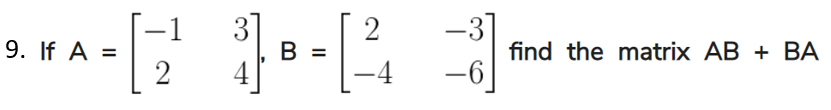Solution: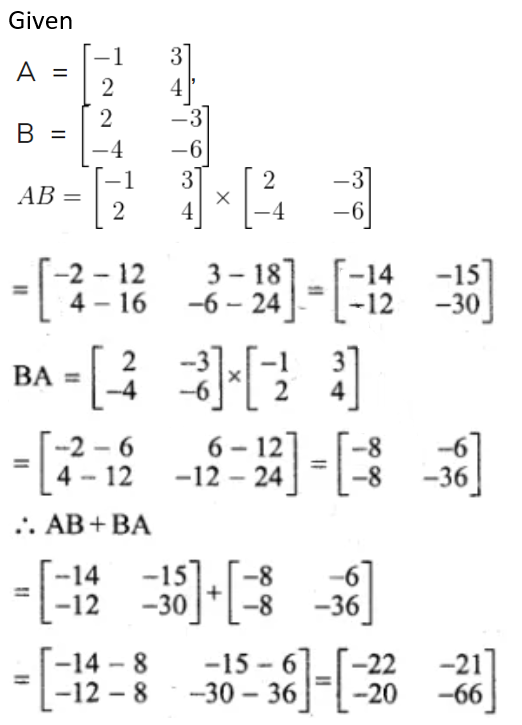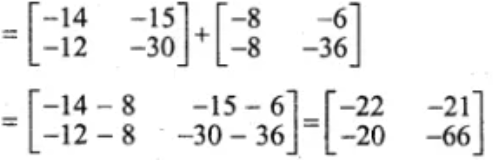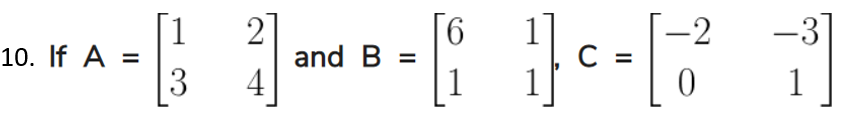Find each of the following and state if they are equal.

(i) CA + B

(ii) A + CB

Solution: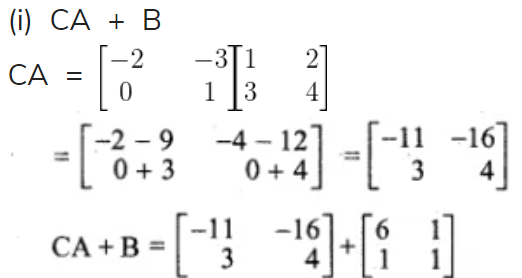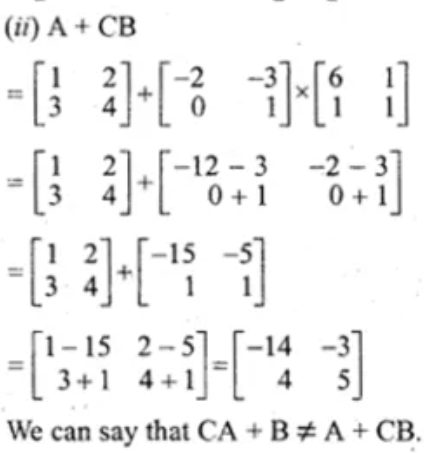We can say that CA + B ≠ A + CB

Chapter test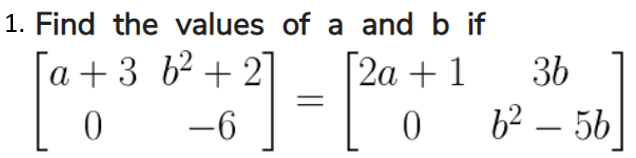Solution:

Given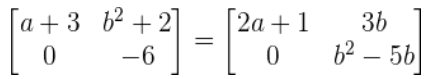comparing the corresponding elements

a + 3 = 2a + 1

⇒ 2a – a =3 – 1

⇒ a = 2 b² + 2 = 3b

⇒ b² – 3b + 2 = 0

⇒ b² – b – 2b + 2 = 0

⇒ b (b – 1) – 2 (b – 1) = 0

⇒ (b – 1) (b – 2) = 0.

Either b – 1 = 0,

then b = 1 or b – 2 = 0,

then b = 2

Hence a = 2, b = 2 or 1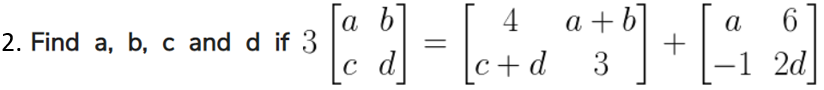Solution: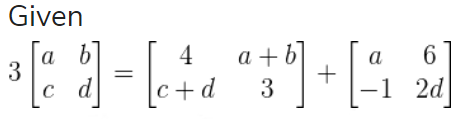Now comparing the corresponding elements

3a = 4 + a

a – a = 4

2a = 4

Therefore, a = 2

3b = a + b + 6

3b – b = 2 + 6

2b = 8

Therefore, b = 4

3d = 3 + 2d

3d – 2d = 3

Therefore, d = 3

3c = c + d – 1

3c – c = 3 – 1

2c = 2

Therefore, c = 1

Hence a = 2,b = 4, c = 1 and d= 3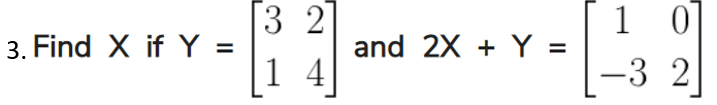Solution: